中山大学 985 双一流 211

Sun Yat-sen University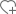关注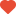已关注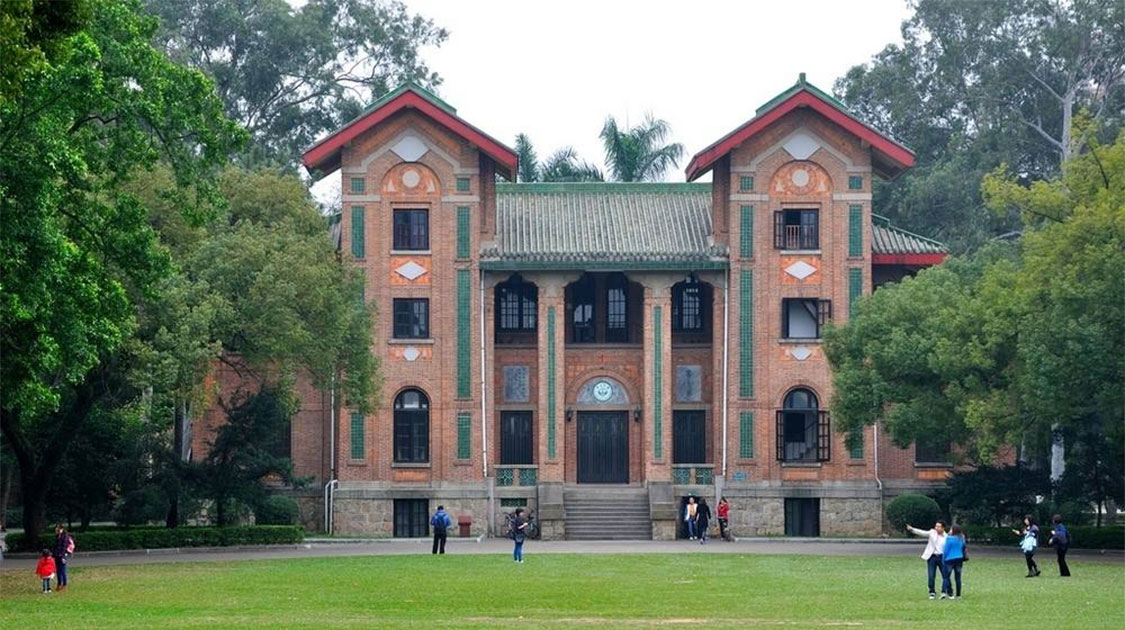•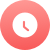1924

•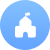公立

•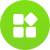综合

•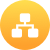教育部

•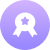本科

•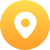广东-广州市

•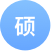59个

•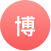49个

院校分数线

多方向招生• 汉语言文学
• 历史学
• 哲学
• 人类学
• 化学
• 法医学
• 护理学
• 行政管理
• 工商管理
• 数学与应用数学
• 网络工程
• 临床医学
• 会计学
• 预防医学
• 微电子学
• 生物科学
• 资源环境与城乡规划管理
• 软件工程
• 生物技术
• 微电子学
• 网络工程
• 临床医学
• 工商管理
• 汉语言文学
• 数学与应用数学
• 化学
• 生物科学
• 资源环境与城乡规划管理
• 软件工程
• 历史学
• 预防医学
• 会计学
• 哲学
• 人类学
• 生物技术
• 法医学
• 行政管理
• 护理学
• 经济学
• 金融学
• 法学
• 汉语言
• 英语语言文学
• 信息与计算科学
• 电子信息科学与技术
• 光信息科学与技术
• 材料化学
• 环境科学
• 计算机科学与技术
• 口腔医学
• 麻醉学
• 药学
• 公共关系学
• 旅游管理
• 图书馆学
• 电子商务
• 物理学
• 应用化学
• 物流管理
• 政治学与行政学
• 自动化
• 市场营销
• 社会工作
• 康复治疗学
• 医学检验学
• 材料物理
• 核能与核技术工程

院系/专业

• 英语（本）
• 日语（本）
• 德语（本）
• 法语（本）

• 经济学（本）
• 管理科学（本）
• 金融学（本）
• 国际商务（本）

• 政治学与行政学（本）
• 社会学（本）
• 汉语言文学（本）
• 哲学（本）
• 法学（本）
• 历史学（本）

• 工商管理（本）
• 会计学（本）

• 人类学（本）
• 考古学（本）
• 社会工作（本）
• 社会学（本）
• 民族学（本）

• 生物技术及应用（本）
• 生物技术（本）
• 生物科学（本）
• 生态学（本）
• 生物工程（本）

• 法学（本）

• 心理学（本）

• 电子科学与技术（本）
• 光学工程（本）
• 信息与通信工程（本）

• 化学（本）
• 材料化学（本）
• 应用化学（本）
• 化学生物学（本）
• 化学工程与工艺（本）
• 高分子材料与工程（本）

• 财政学（本）
• 会计学（本）
• 市场营销（本）
• 经济学（本）
• 物流管理（本）
• 工商管理（本）
• 国际经济与贸易（本）
• 金融学（本）

• （本）

• 麻醉学（本）
• 法医学（本）
• 临床医学（本）
• 生物医学工程（本）
• 医学影像学（本）
• 临床医学(眼科视光方向)（本）
• 医学检验学（本）
• 康复治疗学（本）

• 自然地理与资源环境（本）
• 地理科学（本）
• 人文地理与城乡规划（本）
• 水文与水资源工程（本）
• 地理信息科学（本）
• 城乡规划（本）

• 历史学（本）

• 传播学（本）
• 新闻学（本）

• 卫生检验与检疫（本）
• 预防医学（本）

• 统计学（本）
• 应用统计学（本）
• 数学与应用数学（本）

• 理论与应用力学（本）
• 交通工程（本）

• 软件工程（本）
• 计算机科学与技术（本）
• 信息与计算科学（本）
• 信息安全（本）
• 网络工程（本）

• 汉语言文学（本）

• 材料化学（本）
• 材料物理（本）
• 高分子材料与工程（本）

• 哲学（本）
• 逻辑学（本）

• 环境工程（本）
• 环境科学（本）

• 应用气象学（本）
• 大气科学（本）

• 历史学（本）

• 自动化（本）
• 智能科学与技术（本）

• 行政管理（本）
• 政治学与行政学（本）

• 海洋科学（本）
• 海洋科学（本）

• 护理学（本）

• 国际关学（本）

• 朝鲜语（本）
• 俄语（本）
• 西班牙语（本）
• 英语（本）
• 阿拉伯语（本）

• 物理学（本）
• 光电信息科学与工程(理)（本）

• （本）

• 哲学（本）

• 数学与应用数学（本）
• 信息与计算科学（本）

• 材料物理（本）
• 材料化学（本）

• 会展经济与管理（本）
• 旅游管理（本）

• 药学（本）

• 地质学（本）
• 地球信息科学与技术（本）
• 地质工程（本）

• 物理学（本）
• 天文学（本）

• 汉语言文学（本）

• 化学工程与工艺（本）

• 土木工程（本）

• 电子信息科学与技术（本）
• 通信工程（本）

• 核工程与核技术（本）

• 生物医学工程（本）

• （本）

• （本）

• 海洋工程与技术（本）

大学排名知名校友

•李彦宏

•俞洪敏

•撒贝宁

•马云400-181-5008

(免长话费 工作日 09:00-17:30)